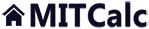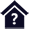#Technical formulas

Clicking on an item from the content will take you to the relevant paragraph.

## Technical formulas

Excel workbook contains solutions to dozens of basic formulas from physics, technology and mechanical engineering. Help, pictures as well as many selection tables with values of various coefficients and material properties are available for the formulas. The following matters are solved in the workbook:

1. Characteristics of solids (volume, surface, weight, inertia moment)
2. Rectilinear motion (speed, acceleration, trajectory, time, force, energy....)
3. Rotation motion (speed, acceleration, trajectory, time, force, energy....)
4. Thermal expansion (longitudinal, cubical)
5. Friction (shear, rolling, journal, bite of rope)
6. Design of engine capacity (pump, blower, hoist, crane)

User interface.

Purchase, Price list.

## Control, structure and syntax of calculations.

Information on the syntax and control of the calculation can be found in the document "Control, structure and syntax of calculations".

### Appendices – This calculation:

Control of this calculation is slightly different from control of ordinary calculations. The sheet “Calculation” contains a single paragraph, where you select the required formula and set values of variables. The selected formula is recalculated and evaluated immediately after a change to any value.

## Process of calculation.

1. Set units to be used in calculation of formulas (SI / Imperial) [1.2]
2. Select the group of formulas you want to work with [1.1]
3. Select the appropriate formula [1.3]
4. In the table of variables, use the switch to mark the variable to be calculated.
5. In the table of variables, set input variables (after a change to a value the result will be recalculated)
6. Add the results to the listing of results using the “Complete listing” button (“Abbreviated listing”)

## Selection of formula, setting of variables. [1.0]

This section is used to select formulas, set variables and evaluate the selected formula. The selected formula is usually provided with help, a picture and in case it is appropriate, also with list boxes with predefined values of some input parameters.

### 1.1 Group of formulas

Select the group (topic) containing the formulas you want to use from the list box. After the group has been selected, the “List of formulas [1.3]” will be completed

### 1.2 Units of calculation

Select the required system of units of calculation from the list box. After the units have been switched over, all values will be immediately recalculated.

### 1.3 List of formulas

Select the formula you want to evaluate from the list. After the selection, the full formula including help is shown on line [1.5]. All variables contained in the selected formula are specified in the rectangular area. Help for the selected formula is placed as a note next to the formula on line [1.5] and it can be displayed by moving the cursor over the line number.

### Area of variables

Individual variables contained in the selected formula are shown in the rectangular area of variables (max. 10). One line is designed for each formula; it shows:

• Name of variable
• Identifier of variable in the formula
• Value of variable
• Switch specifying which variable is resultant
• Units

Variables are provided with a defined range of permitted input values. In case toy attempt to set a value outside the permitted range, a warning message is shown.

### Picture, Predefined value

On line [1.6], there is a switch (Picture / Tables). It is used to switch between the display of a picture or list boxes containing predefined values and coefficients of some variables. The picture or list boxes are shown to the right of the area of variables.

### Starting calculator

In the heading of the "Calculation" sheet there is "Start calculator" button. Press it to start (show) the standard Windows calculator. If you want to launch another program, use the "Options" sheet to set its parameters.

## Listing of results 

In this paragraph, after the "Abbreviated listing" ("Complete listing") button has been pressed, the results of the calculation of the formula are displayed, including input values, the formula itself and in case of "Complete listing", descriptions of individual variables. Buttons used to add the listing of the current formula are placed on line [1.6]. Press "Clear" to remove all records from the paragraph "Listing of results".

## List of formula groups

This chapter specifies the basic list of formula groups.

### 3D Solids (prism, pyramid, cone, cylinder, sphere…)

Formulas for calculating volume, surface, weight and inertia moment of selected 3D objects.

#### Calculation of volume and surface

For the calculation, set the dimensions of a solid. The surface calculation is additional and cannot be used for back calculation of dimensions

#### Calculation of inertia moment and weight

Inertia moment is calculated with respect to the axis marked with a bold dashed-and-dotted line in the picture.

### Rectilinear motion (speed, trajectory, time, force, energy…)

Formulas for calculating various physical properties of kinetics and dynamics of a primary element (solid) in rectilinear motion.

### Rotation motion (speed, trajectory, time, force, energy…)

Formulas for calculating various physical properties of kinematics and dynamics of a primary element (solid) in motion in a circle (rotation motion).

### Friction (shear, rolling, journal, bite of rope)

#### Shear friction

During steady rectilinear motion of a solid on a rough plain or at the point of rest and motion, the friction force given by the empirical (Coulomb) law F = Q * f, with Q for normal stress and f for non-dimensional coefficient of shear friction, acts against the driving force. It is mainly the function of speed. For solid friction it can be considered a constant.

#### Journal friction

It originates at points of rotation bearing of solids (radial, axial journal). It arises as a moment of journal friction M in the opposite direction of rotation. In case of an axial bearing in a worn-in bearing, we consider the distribution of pressure according to the law of equiaxed hyperbola; in case of a non worn-in bearing, we consider the specific pressure is distributed evenly on the bearing surface.

#### Friction of belts and ropes

Bite of rope of flexible fibre on the surface of a solid arises as the difference of tensile forces F1 <> F2 as a result of shear friction between the belt and the solid, for which the Euler relation applies.

#### Rolling friction (resistance)

It originates during rolling of a solid on a flat surface as a result of deformations in the place of contact and it is expressed by the shift of normal reaction force by the so-called lever arm of rolling resistance in the direction of rolling. The lever arm of rolling resistance is ascertained in an experimental way for different materials and also depends on the rolling radius.

For the motion of a system (truck, car...), the relationship of the force required to keep the system in motion to the total normal force is ascertained. This so-called traction coefficient includes resistance of rolling, shear, journal and other frictions.

### Thermal expansion (longitudinal, cubical)

Coefficients of thermal expansion are specified for normal conditions at 20°C (68°F). If you are solving expansion in the area of high or low temperatures, consult technical literature for the appropriate coefficients of thermal expansion.

### Design of engine capacity (pump, blower, hoist, crane)

These formulas enable fast preliminary calculation/design of engine capacity for many standard types of machinery.

#### Input of pump engine

Total efficiency of a pump drive usually ranges between 0.50 ~ 0.70 (50% - 70%)

#### Input of blower engine

Total efficiency of a blower (compressor) drive usually ranges between 0.50 ~ 0.70 (50% - 70%)

#### Input of hoist (crane) engine

In case of a personnel hoist, a weight of 75kg / 165lb per one person is considered. If the hoist is equipped with a counterweight, a half-load is considered.
Total efficiency of the drive ranges falls between 0.50 ~ 0.70 (50% - 70%)

###### Example:

For a personnel hoist for 10 people with counterweight, the load is:
10 x 75kg = 750kg / 2 = 375kg
10 x 165lb = 1650lb / 2 = 825lb

## Setting calculations, change the language.

Information on setting of calculation parameters and setting of the language can be found in the document "Setting calculations, change the language".

## Workbook modifications (calculation).

General information on how to modify and extend calculation workbooks is mentioned in the document "Workbook (calculation) modifications".

### Extension with user formulas

You can extend the workbook with the evaluation of often-used formulas. You can add new formulas in the area (chapter) designed for them. You will find the appropriate table in "Tables" named "User formulas (T_UserFormulas)".

In the table, complete the following columns:

 Column Data to complete 2 Name of formula 3 Formula to be displayed 10-19 Evaluating formula for individual variables "_V1", "_V2"... in the format _V1 = f(_V2,_V3....); _V2 = f (_V1,_V3....) 20 Name of variable _V1 21 Identification of variable _V1 22 Initial value of variable _V1 23 Units of variable _V1 24 Conversion coefficient between SI and Imperial units 25-26 Range of permitted values for _V1 27 Name of table containing default values to be selected from list box 30 Name of variable _V2 ... ...

### Calculator Settings

You can start a random program using the "Start calculator” button on the sheet "Calculation" (standard Windows calculator is default). You can set parameters in "Settings".

#### 1.2 Name of program (calculator)

Set the name of the target program, or set the name of the file including the path in case the path to the file is not set in system variable "PATH".

#### 1.3 Name of program (calculator) window

In order to prevent an already running program restarting, but have it show in the foreground, set the name of the program window.